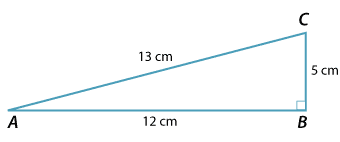### Values of the trigonometric ratios

For triangle ABC:Detailed description

\begin{align}\text{sin} \ \theta &= \dfrac{5}{13}\\ \text{cos} \ \theta &= \dfrac{12}{13}\\ \text{tan} \ \theta &= \dfrac{5}{12}\end{align}

### Using a calculator

Given any angle $$\theta$$, the values of sin $$\theta$$, cos $$\theta$$ and tan $$\theta$$ can be found using a calculator:

sin 30° = 0.5

cos 30° ≈ 0.8660 (correct to 4 decimal places)

tan 30° ≈ 0.5774 (correct to 4 decimal places)

Because the hypotenuse is the longest side of the triangle, the sine and cosine ratios are always less than 1.

0 < sin $$\theta$$ < 1 and 0 < cos $$\theta$$ < 1